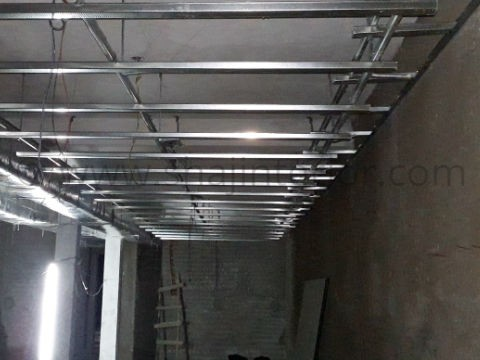# How to calculate gypsum false ceiling material?## How to calculate gypsum false ceiling material?

In this post you will learn how to calculate gypsum false ceiling materials for a 10'x10' size of room. There are two types of installation method exist in the current market. However we are going to calculate the false ceiling materials for a 100 sq.ft (10'x10') of plain false ceiling as per the local false ceiling contractor’s installation method which is most common today.

### Material Calculation Method

• Ceiling Angle (12'): Total sq.ft ÷ 16 x ceiling drop length ÷ channel length (i.e. 100 ÷ 24 x 9" ÷ 12' = 0.52)
• Perimeter Channel (12'): Total wall length ÷ 11.5 (i.e. 40 ÷ 12' = 3.48)
• Intermediate Channel (12'): Total sq.ft ÷ 64 (i.e. 100 ÷ 64 = 1.56)
• Ceiling Section (12'): Total sq.ft ÷ 72 (i.e. 100 ÷ 28 = 3.57)
• Plaster Board (6'x4'): Total sq.ft ÷ 24 (i.e. 100 ÷ 28 = 4.17)

### Required Key Materials List

1. Ceiling Angle: 1 No
2. Perimeter Channel: 4 No's
3. Intermediate Channel: 2 No's
4. Ceiling Section: 4 No's
5. Plasterboard: 5 No's